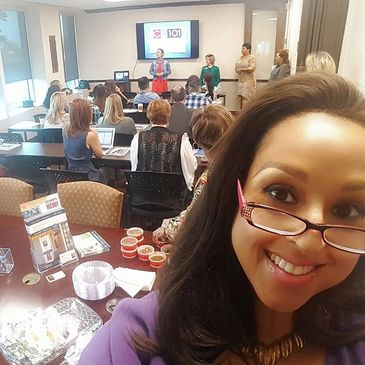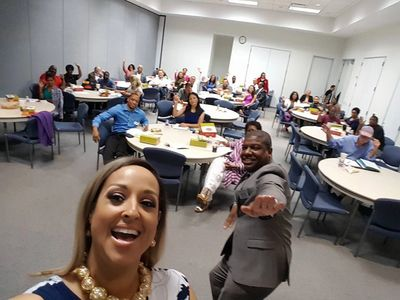learning is earning learning is earning learning is earning learning is earning learning is earning learning is earning learning is earning learning is earning learning is earning learning is earning learning is earning learning is earning learning is earning learning is earning learning is earning learning is earning learning is earning learning is earning learning is earning learning is earning learning is earning learning is earning learning is earning learning is earning learning is earning learning is earning learning is earning learning is earning learning is earning learning is earning learning is earning learning is earning learning is earning learning is earning learning is earning learning is earning learning is earning learning is earning learning is earning learning is earning learning is earning learning is earning learning is earning learning is earning learning is earning learning is earning learning is earning learning is earning learning is earning learning is earning learning is earning learning is earning learning is earning learning is earning learning is earning learning is earning learning is earning learning is earning learning is earning learning is earning learning is earning learning is earning learning is earning learning is earning learning is earning learning is earning learning is earning learning is earning learning is earning learning is earning learning is earning learning is earning learning is earning learning is earning learning is earning learning is earning learning is earning learning is earning learning is earning learning is earning

# Teaching the basics & beyond of commercial leasing transactions!

Welcome to
The Guillory Group School of Real Estate
Texas' ONLY Commercial Leasing-Focused Real Estate School

learning is earning learning is earning learning is earning learning is earning learning is earning learning is earning learning is earning learning is earning learning is earning learning is earning learning is earning learning is earning learning is earning learning is earning learning is earning learning is earning learning is earning learning is earning learning is earning learning is earning learning is earning learning is earning learning is earning learning is earning learning is earning learning is earning learning is earning learning is earning learning is earning learning is earning learning is earning learning is earning learning is earning learning is earning learning is earning learning is earning learning is earning learning is earning learning is earning learning is earning learning is earning learning is earning learning is earning learning is earning learning is earning learning is earning learning is earning learning is earning learning is earning learning is earning learning is earning learning is earning learning is earning learning is earning learning is earning learning is earning learning is earning learning is earning learning is earning learning is earning learning is earning learning is earning learning is earning learning is earning learning is earning learning is earning learning is earning learning is earning learning is earning learning is earning learning is earning learning is earning learning is earning learning is earning learning is earning learning is earning learning is earning learning is earning learning is earning learning is earning

# Teaching the basics & beyond of commercial leasing transactions!

Welcome to
The Guillory Group School of Real Estate
Texas' ONLY Commercial Leasing-Focused Real Estate School

## Guillory Group School of Real Estate

#### We Come to You!We teach classes all over Texas -- in title offices, private brokerages, organizational luncheons and live via the Internet. While we're based right here in Houston, yet we've traveled to Austin and San Antonio, Tomball, Galveston and every place in between. Did we mention that a complimentary lunch is almost always included? The 2019 schedule already has classes booked through November. Did you book yours?

#### We're a Texas original!There are some great real estate schools in Texas, with the largest being woman-owned and that's phenomenal in our eyes! And while we're also woman-owned, that's not what makes our school unique. What's different about us is our laser focus on teaching the basics and beyond of commercial real estate. We've been in business since 2012 and have been growing strong every year. Thank you, Texas, for the love!

#### We Are Focused!While we'd love to offer you courses in every area of real estate, we limit what we do so we can do it better than anyone else. So, if you're looking to obtain a real estate license, we can tell ya about some great institutions. But if you're a broker or agent, a tenant or even a landlord looking to expand your knowledge of commercial real estate transactions, this is your one-stop shop for education and information.

## Our Services#### real estate classes

We have a variety of courses to choose from, all centered on some aspect of commercial leasing transactions. Our classes can be combines in full-day boot camps or taken separately. Visit our Registration page to view our calendar.#### private consultation

Sometimes, brokers and agents need transactional guidance NOW and simply cannot wait for a class! We're still here to help you whether this is your first commercial transaction or your 21st. Just give us a call or send an e-mail...and ask for owner Michica 'Mish' Guillory - Broker, GRI, ITI, TRLP.